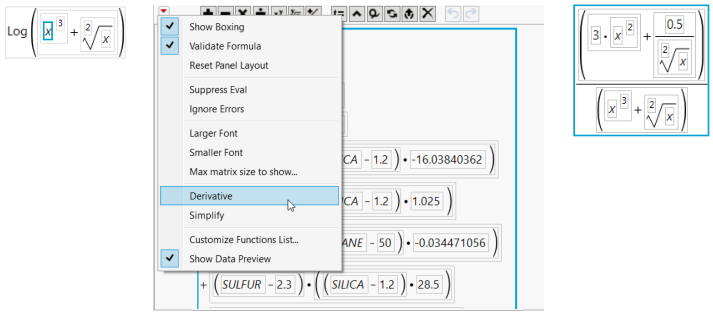Publication date: 08/13/2020

## Calculate Derivatives

The JMP Formula Editor can find and display the derivative of a function. The derivative is found with respect to the function argument (a single variable name) you highlight. Therefore, in order to differentiate with respect to x, x must be one of the arguments in the expression. The red triangle menu next to the keypad contains the Derivative command.

To calculate a derivative, follow these steps:

1. Enter a function.

2. Highlight a variable.

3. Select Derivative from the menu.

Figure 7.15 Using the Derivative Option